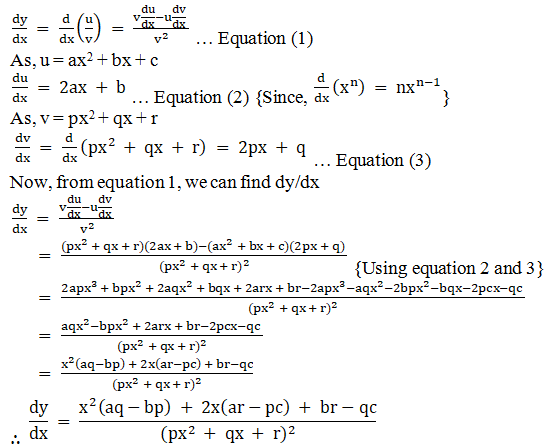# RD Sharma Solutions for Class 11 Chapter 30 - Derivatives Exercise 30.5

This exercise deals with fundamental rules for differentiation which is based on the quotient rule for differentiation. Students can practice the problems on a regular basis and clear their doubts pertaining to the subject by referring to the solutions. The solutions are designed strictly according to the current syllabus and marks weightage in the annual exam. Students can boost their confidence level by solving tricky problems with the help of solutions. RD Sharma Class 11 Maths Solutions pdf is available here, students can download the same from the links provided below.

## Download the pdf of RD Sharma Solutions for Class 11 Maths Exercise 30.5 Chapter 30 – Derivatives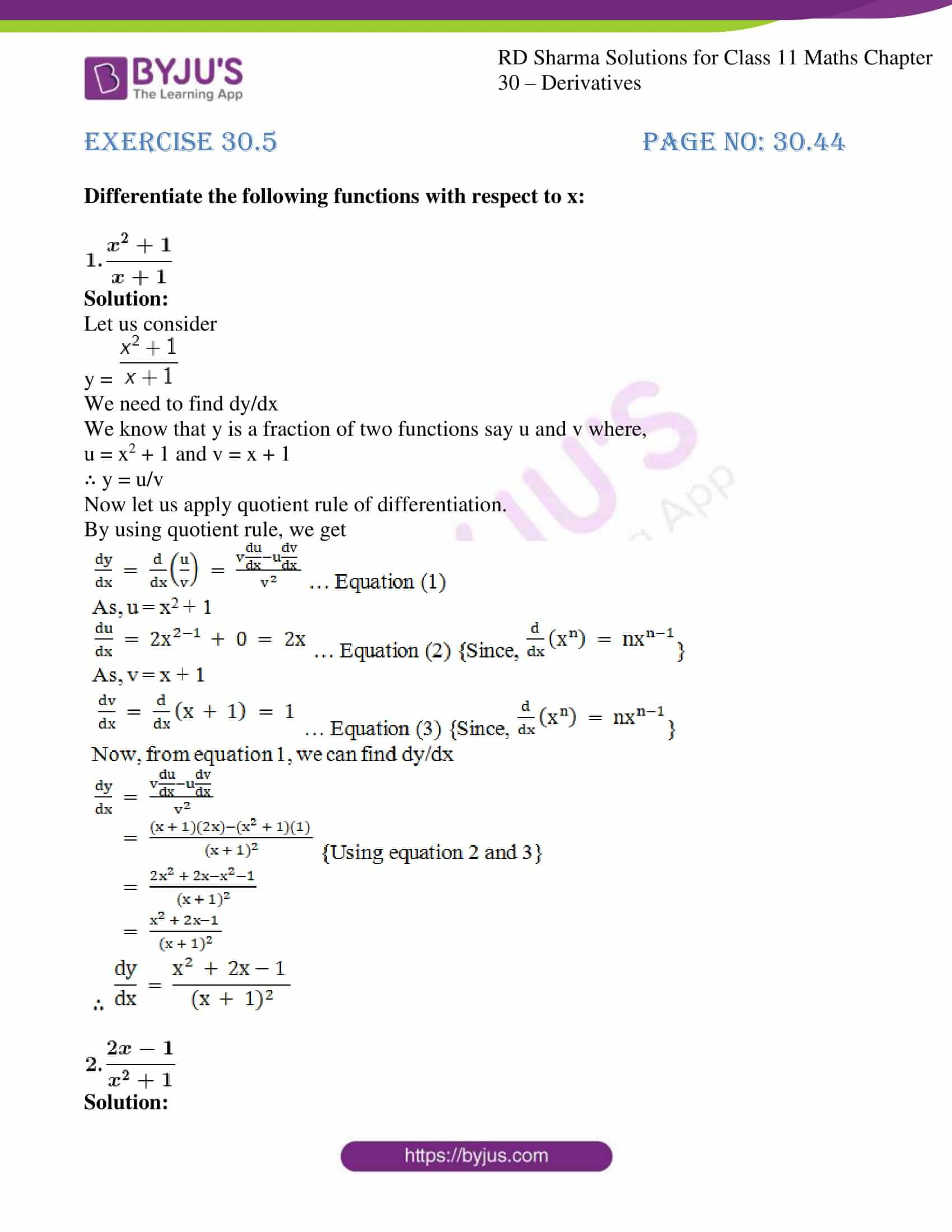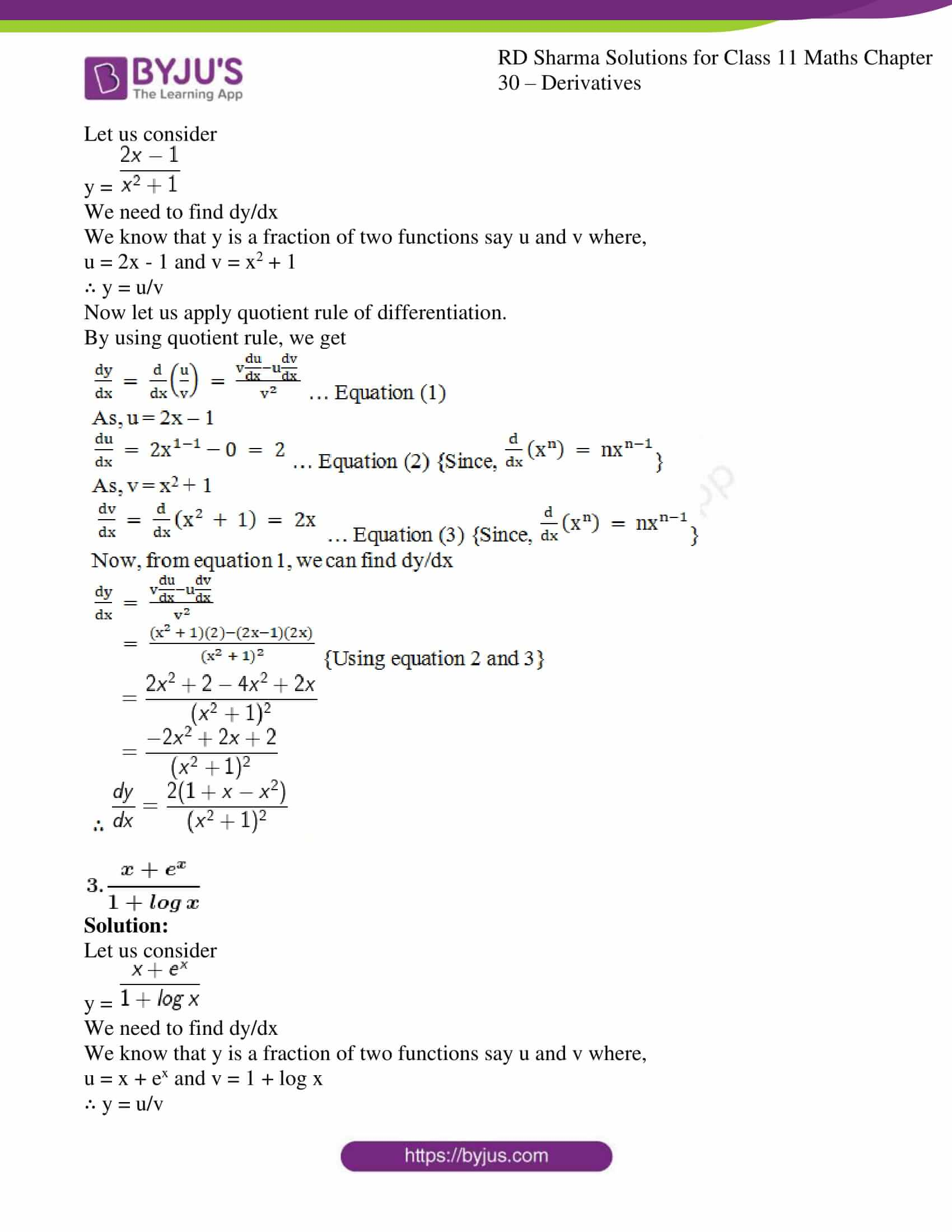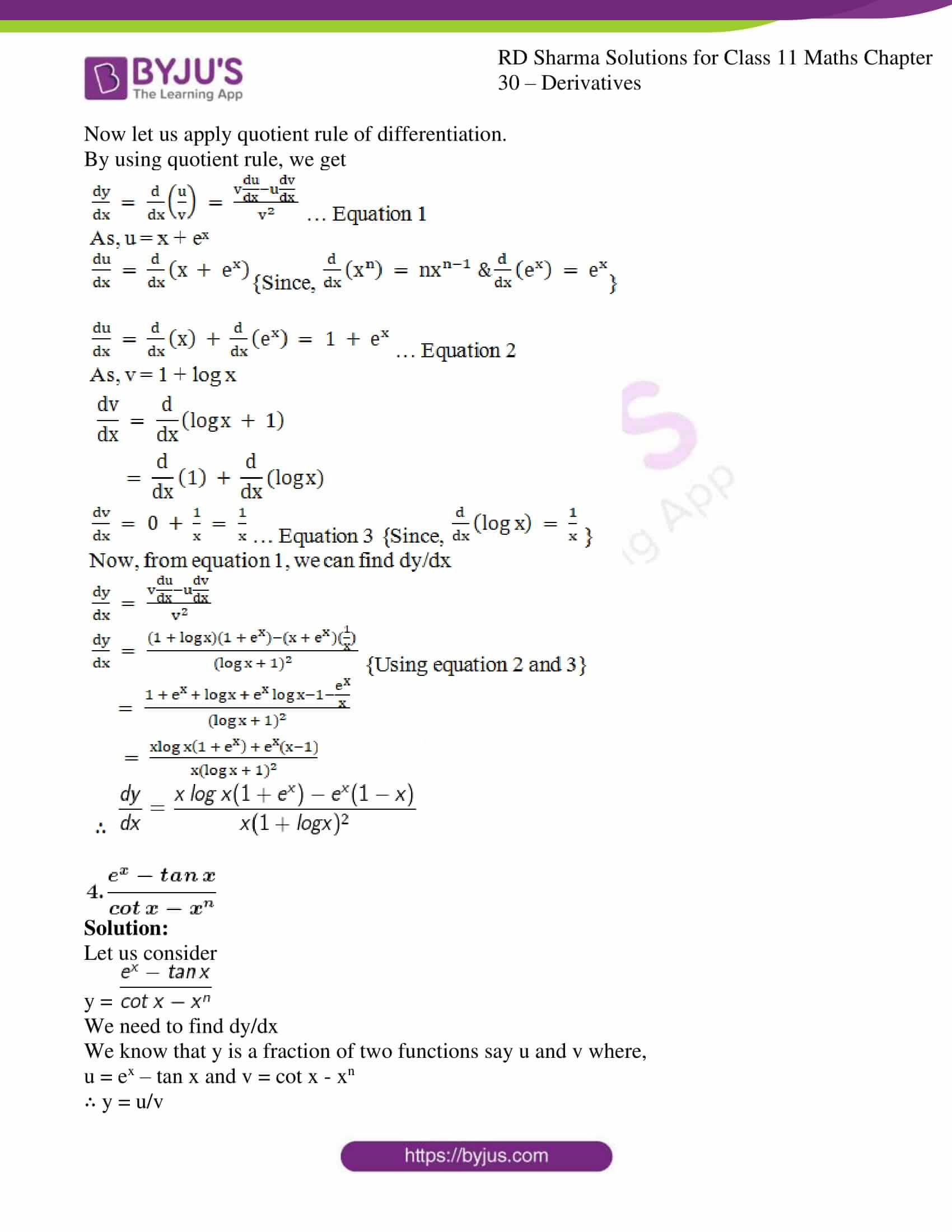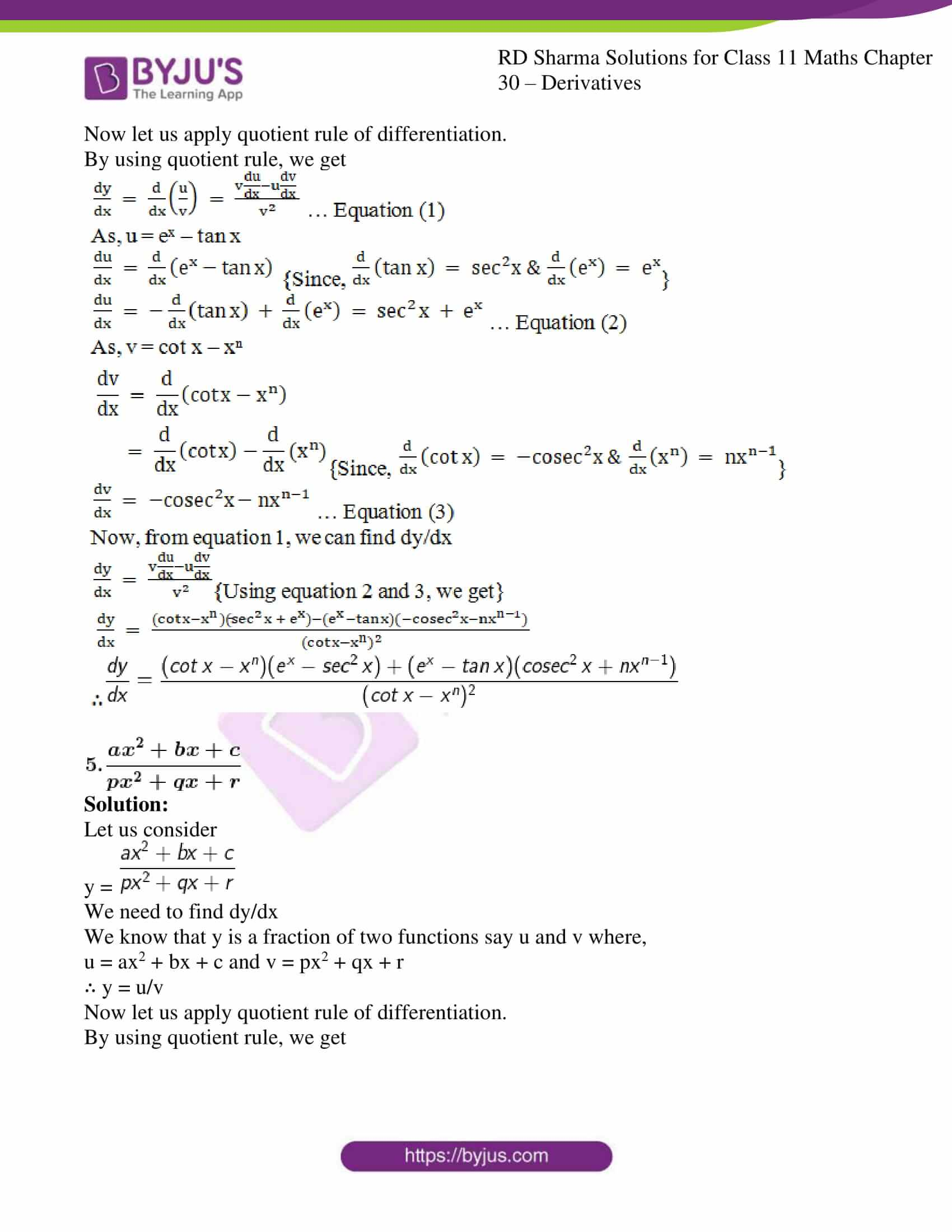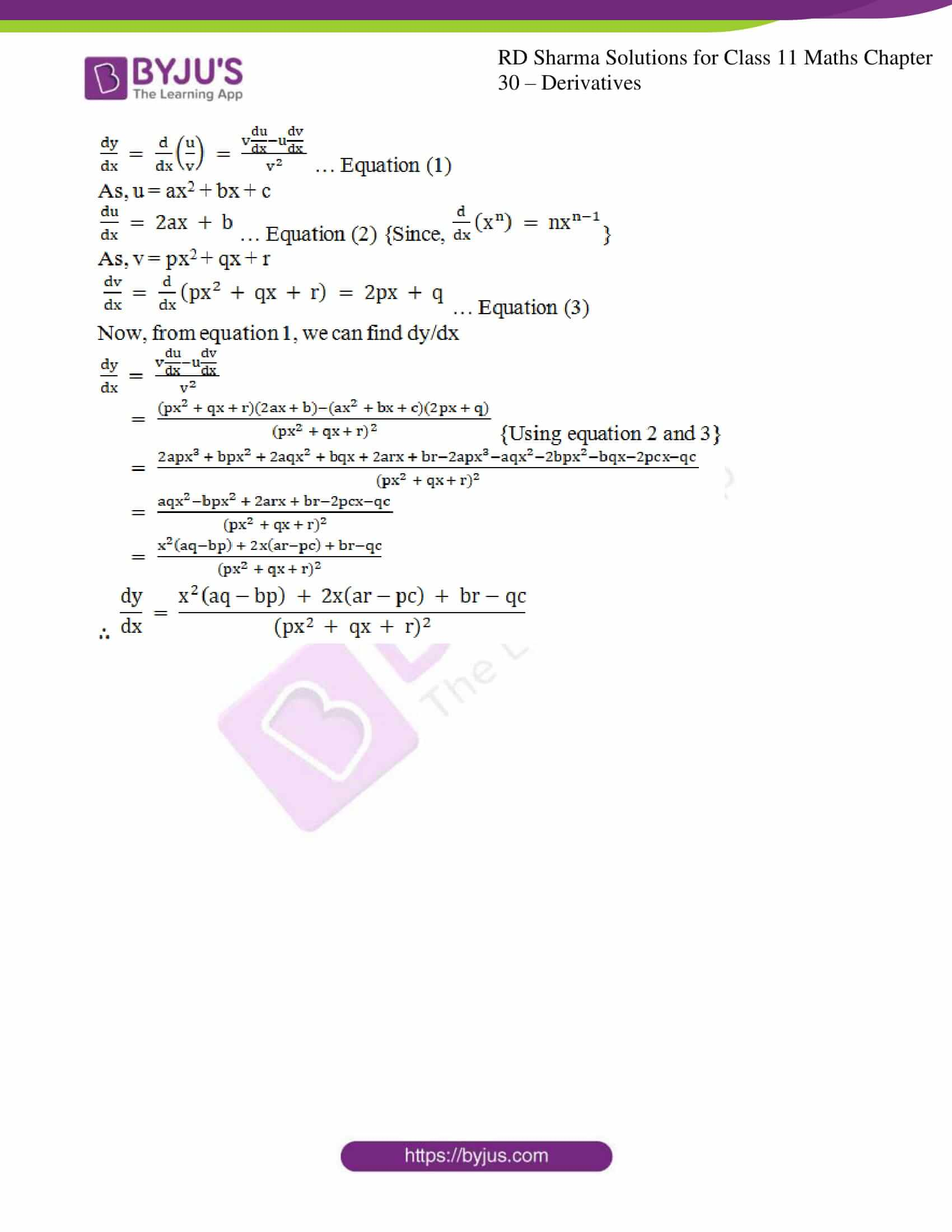### Access answers to RD Sharma Solutions for Class 11 Maths Exercise 30.5 Chapter 30 – Derivatives

#### EXERCISE 30.5 PAGE NO: 30.44

Differentiate the following functions with respect to x: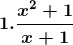Solution:

Let us consider

y =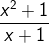We need to find dy/dx

We know that y is a fraction of two functions say u and v where,

u = x2 + 1 and v = x + 1

∴ y = u/v

Now let us apply quotient rule of differentiation.

By using quotient rule, we get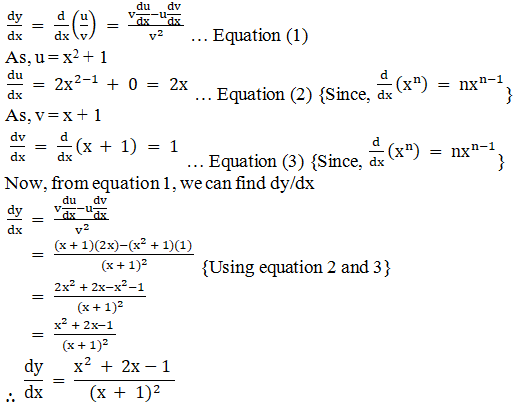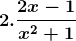Solution:

Let us consider

y =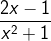We need to find dy/dx

We know that y is a fraction of two functions say u and v where,

u = 2x – 1 and v = x2 + 1

∴ y = u/v

Now let us apply quotient rule of differentiation.

By using quotient rule, we get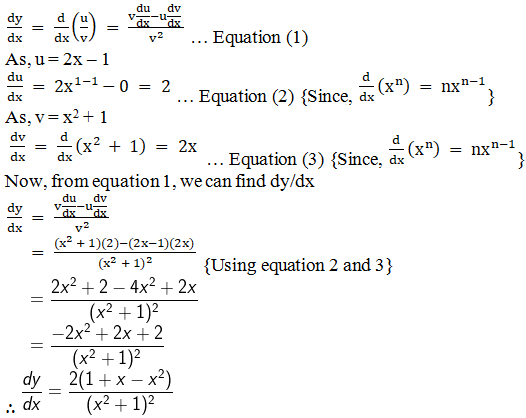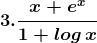Solution:

Let us consider

y =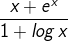We need to find dy/dx

We know that y is a fraction of two functions say u and v where,

u = x + ex and v = 1 + log x

∴ y = u/v

Now let us apply quotient rule of differentiation.

By using quotient rule, we get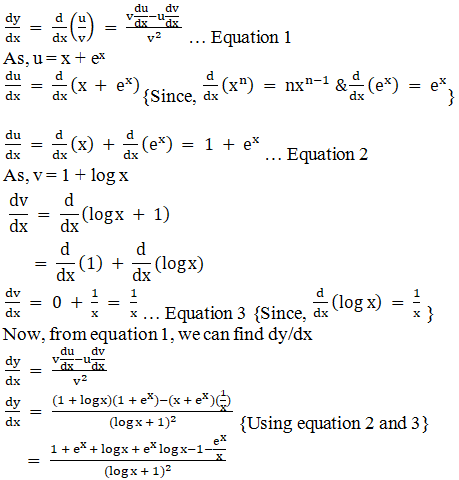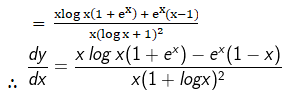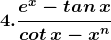Solution:

Let us consider

y =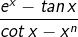We need to find dy/dx

We know that y is a fraction of two functions say u and v where,

u = ex – tan x and v = cot x – xn

∴ y = u/v

Now let us apply quotient rule of differentiation.

By using quotient rule, we get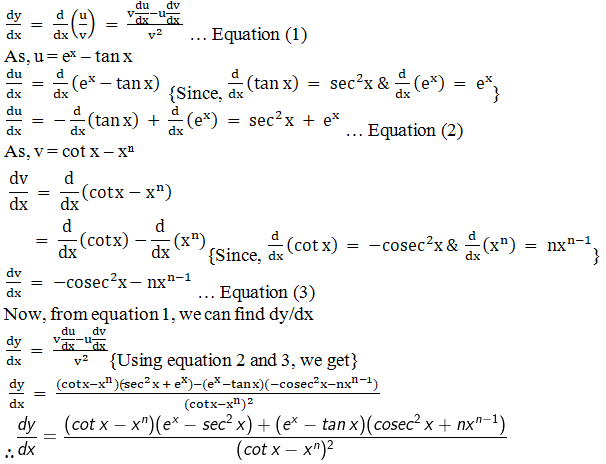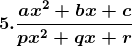Solution:

Let us consider

y =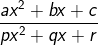We need to find dy/dx

We know that y is a fraction of two functions say u and v where,

u = ax2 + bx + c and v = px2 + qx + r

∴ y = u/v

Now let us apply quotient rule of differentiation.

By using quotient rule, we get